# Identity (mathematics) facts for kids

Kids Encyclopedia Facts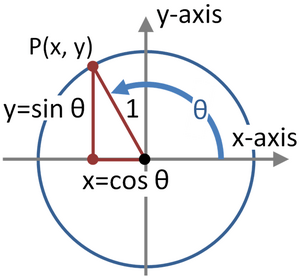Visual proof of the Pythagorean identity. For any angle θ, The point (cos(θ),sin(θ)) lies on the unit circle, which satisfies the equation x2+y2=1. Thus, cos2(θ)+sin2(θ)=1

In mathematics, the term identity has several important uses:

• An identity is an equality that remains true even if you change all the variables that are used in that equality.

An equality in mathematical sense is only true under more particular conditions. For this, the symbol ≡ is sometimes used. (However, this can lead to misunderstandings since the same symbol can also be used for a congruence relation.)

• In algebra, an identity or identity element of a set S with a binary operation is an element e which combined with any element s of S produces s.
• The identity function from a set S to itself, often denoted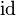$\mathrm{id}$ or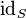$\mathrm{id}_S$, such that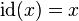$\mathrm{id}(x)=x$ for all x in S.
• In linear algebra, the identity matrix of size n is the n-by-n square matrix with ones on the main diagonal and zeros elsewhere.

## Examples

### Identity relation

A common example of the first meaning is the trigonometric identity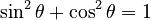$\sin ^2 \theta + \cos ^2 \theta = 1\,$

which is true for all real values of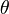$\theta$ (since the real numbers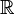$\Bbb{R}$ are the domain of sin and cos), as opposed to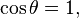$\cos \theta = 1,\,$

which is true only for values of$\theta$ in a subset of the domain.

### Identity element

The concepts of "additive identity" and "multiplicative identity" are central to the Peano axioms. The number 0 is the "additive identity" for integers, real numbers, and complex numbers. For the real numbers, for all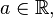$a\in\Bbb{R},$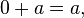$0 + a = a,\,$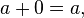$a + 0 = a,\,$ and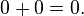$0 + 0 = 0.\,$

Similarly, The number 1 is the "multiplicative identity" for integers, real numbers, and complex numbers. For the real numbers, for all$a\in\Bbb{R},$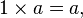$1 \times a = a,\,$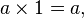$a \times 1 = a,\,$ and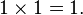$1 \times 1 = 1.\,$

### Identity function

A common example of an identity function is the identity permutation, which sends each element of the set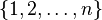$\{ 1, 2, \ldots, n \}$ to itself.

## Comparison

These meanings are not mutually exclusive; for instance, the identity permutation is the identity element in the set of permutations of$\{ 1, 2, \ldots, n \}$ under composition.Identity (mathematics) Facts for Kids. Kiddle Encyclopedia.